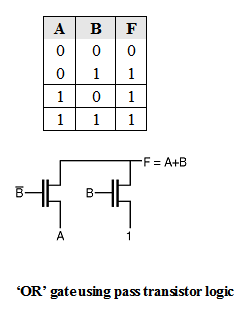Home > Digital CMOS Design > Pass Transistor Logic > OR gate using pass transistor logic

OR-gate-using-pass-transistor-logic | Pass-Transistor-Logic

'OR' gate using pass transistor logic

The truth table of 'OR' gate is as shown in Table below. Figure below shows the implementation of OR function using NMOS transistors only. In this gate if the B input is high the right NMOS is turned ON and copies logic 1 to F and this operation does not affected by 'A' input. When B is low the left NMOS is turned ON the logic of 'A' is copied to the output F.OR gate implemented using transmission gate:

Figure below shows the OR gate implemented using transmission gate. The input variables are controlling the pMOS and transmission gate. The upper branch passes data when

A = 1, and transmission gate passes B when A = 0.